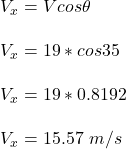## A 1-kg discus is thrown with a vertical velocity of 19 m/s at an angle of 35 degrees from a height of 1.94 m. Do not factor in air resistanc

Question

A 1-kg discus is thrown with a vertical velocity of 19 m/s at an angle of 35 degrees from a height of 1.94 m. Do not factor in air resistance. Calculate the vertical and horizontal velocities.

in progress 0
6 months 2021-07-31T19:55:22+00:00 1 Answers 12 views 0

## Answers ( )

Vertical velocity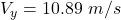Horizontal velocity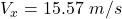Explanation:

If a 1 -kg is thrown vertically with a velocity of 19  m/s at an angle of 35 °C from a height 1.94 m

The vertical and horizontal component can be resolved as: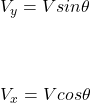For Vertical component :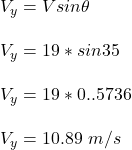For horizontal velocity